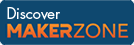Cody

# Problem 44350. Breaking Out of the Matrix

Created by James in Community

Do you want to take the Red Pill, or the Blue Pill?

If you take the Blue Pill, you will simply pass along to the next problem, not knowing what Cody has in store for you.

If you take the Red Pill, you will be asked to write a MATLAB function that will Break a Matrix. The inputs to the function will be a matrix M, along with a number of rows (R) and columns (C). You goal is to break the larger 2-D matrix up into a 3-D matrix comprised of enough RxC matrices so that you can recreate the 2-D matrix. When creating your 3-D matrix, go down the columns first, and then across the rows. Increment only one column (or one row) at a time. Do not go C columns down at each step.

For example, R=2 and C=3, and M is as follows:

``` M=[1 4 7 10
2 5 8 11
3 6 9 12]```

This means that your output should be a 2x3x4 matrix:

``` X(:,:,1) =
1     4     7
2     5     8
X(:,:,2) =
2     5     8
3     6     9
X(:,:,3) =
4     7    10
5     8    11
X(:,:,4) =
5     8    11
6     9    12```

You can assume that R and C will always be less than or equal to the appropriate dimension of the original matrix. Good luck!

### Solution Stats

40.4% Correct | 59.6% Incorrect
Last solution submitted on Mar 08, 2019

#### TagsMATLAB and Simulink resources for Arduino, LEGO, and Raspberry Pi